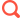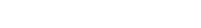# 帝国cms实现产品参数等多条件存储并检索的实现方案1. <script type="text/javascript" src="/scripts/jquery.js"></script>
2. <span id="rongliangcon">
3. <?php
4. \$r[rongliang]=trim(\$r[rongliang],"|");
5. \$morefr=explode('|',\$r[rongliang]);
6. \$mfcount=count(\$morefr);
7. for(\$mfi=0;\$mfi<\$mfcount;\$mfi++)
8. {
9. ?>
10. <input name="rongliang[]" type="text" value="<?=\$morefr[\$mfi]?>" size="">
11. <?php
12. }
13. if(!\$r[rongliang]){
14. ?>
15. <input name="rongliang[]" type="text" value="" size="">
16. <?php
17. }
18. ?>
19. </span>
21. <script>
23.     \$("#rongliangcon").append('<input name="rongliang[]" type="text" value="" size=""> ');
24. }
25. </script>3.     \$rlcon='|';
4.     for(\$i=0;\$i<count(\$rongliang);\$i++){
5.         \$rlcon.=\$rongliang[\$i];
6.     }
8. }

1. if(\$andr[\$i]=='rongliang'){
2.     \$listandf.=\$doandor.\$andr[\$i]." like '%|".\$andval."|%'";
3. }Tags： 多条件   存储   检索

### 相关推荐